Test: Electromagnetics - 2

# Test: Electromagnetics - 2

Test Description

## 20 Questions MCQ Test GATE ECE (Electronics) 2023 Mock Test Series | Test: Electromagnetics - 2

Test: Electromagnetics - 2 for Electronics and Communication Engineering (ECE) 2023 is part of GATE ECE (Electronics) 2023 Mock Test Series preparation. The Test: Electromagnetics - 2 questions and answers have been prepared according to the Electronics and Communication Engineering (ECE) exam syllabus.The Test: Electromagnetics - 2 MCQs are made for Electronics and Communication Engineering (ECE) 2023 Exam. Find important definitions, questions, notes, meanings, examples, exercises, MCQs and online tests for Test: Electromagnetics - 2 below.
Solutions of Test: Electromagnetics - 2 questions in English are available as part of our GATE ECE (Electronics) 2023 Mock Test Series for Electronics and Communication Engineering (ECE) & Test: Electromagnetics - 2 solutions in Hindi for GATE ECE (Electronics) 2023 Mock Test Series course. Download more important topics, notes, lectures and mock test series for Electronics and Communication Engineering (ECE) Exam by signing up for free. Attempt Test: Electromagnetics - 2 | 20 questions in 60 minutes | Mock test for Electronics and Communication Engineering (ECE) preparation | Free important questions MCQ to study GATE ECE (Electronics) 2023 Mock Test Series for Electronics and Communication Engineering (ECE) Exam | Download free PDF with solutions
 1 Crore+ students have signed up on EduRev. Have you?
Test: Electromagnetics - 2 - Question 1

### The locus of the resistance 50 Q such that Zo=50Ω for a constructing a smith chart is

Detailed Solution for Test: Electromagnetics - 2 - Question 1

Radius of the normalized resistance, Centre  =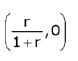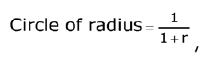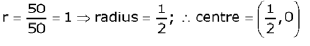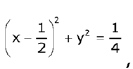Radius = 1/2 and Centre = (1/2, 0)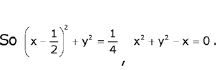Test: Electromagnetics - 2 - Question 2

### A distortion less transmission line hasZ0 = 50Ω,α = 10mNP/m,u = 0.167C (C = 3x 108m/s'), then R and L of transmission line are

Detailed Solution for Test: Electromagnetics - 2 - Question 2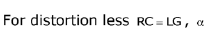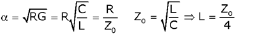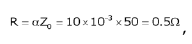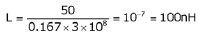Test: Electromagnetics - 2 - Question 3

### If PSWR is power standing wave ratio, then VSWR and PSWR corresponding to r = 0.5∠450 is

Detailed Solution for Test: Electromagnetics - 2 - Question 3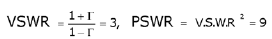Test: Electromagnetics - 2 - Question 4

Z0 = 600Ω, VSWR = 1.5, the distance at which first maximum occurs is 20cm from the load. If the operating frequency of the transmission line is 300 MHz, then the reflection coefficient is given by

Detailed Solution for Test: Electromagnetics - 2 - Question 4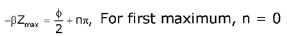For first maximum, n = 0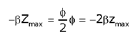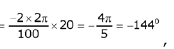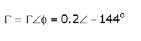Test: Electromagnetics - 2 - Question 5

For the transmission line shown below, the voltage which appears at the load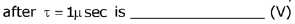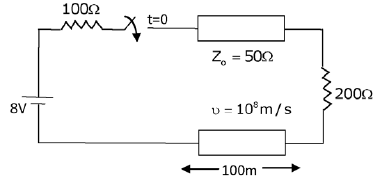Detailed Solution for Test: Electromagnetics - 2 - Question 5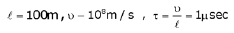All appears at the load. Including this there will some part which is reflected back From the load we should include thisf.Test: Electromagnetics - 2 - Question 6

A transmission line having a characteristic impedance of 300fl is feeding a dipole antenna. Frequency of operation is 100 MHz and length of dipole antenna is 1.5m.

Q.

Magnitude of reflection coefficient on the line is

Detailed Solution for Test: Electromagnetics - 2 - Question 6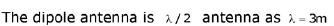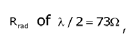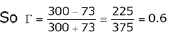Test: Electromagnetics - 2 - Question 7

A transmission line having a characteristic impedance of 300fl is feeding a dipole antenna. Frequency of operation is 100 MHz and length of dipole antenna is 1.5m.

Q.

If Pout from the transmission line is 100 watts, then the power tramsmitted by the antenna is

Detailed Solution for Test: Electromagnetics - 2 - Question 7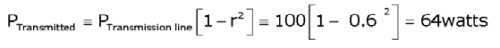Test: Electromagnetics - 2 - Question 8

Consider the impedance matching series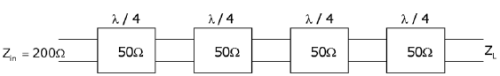Q.

The load impedance ZL is given by

Test: Electromagnetics - 2 - Question 9

Consider the impedance matching seriesQ.

The reflection coefficient at the load end is

Detailed Solution for Test: Electromagnetics - 2 - Question 9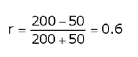Test: Electromagnetics - 2 - Question 10

A uniform plane wave is incident normally on a dielectric interface with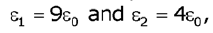the current reflection coefficient, current transmission, current standing wave ration (ISWR) are

Detailed Solution for Test: Electromagnetics - 2 - Question 10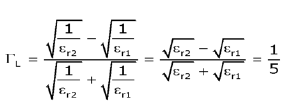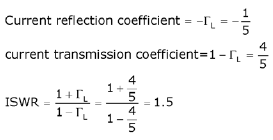Test: Electromagnetics - 2 - Question 11

Match the following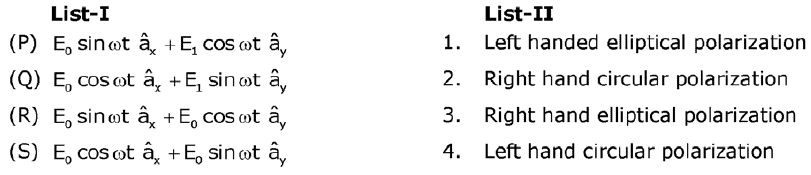Detailed Solution for Test: Electromagnetics - 2 - Question 11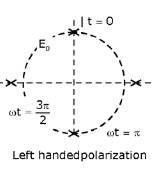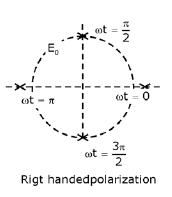If the amplitudes are equal, then it is circularly polarized otherwise elliptical polarization.

Test: Electromagnetics - 2 - Question 12

In air, a loss less transmission line of length 50 cm with L=10μH/mand C=40 pF/m is operated at 25 MHz. Its electrical path length is

Detailed Solution for Test: Electromagnetics - 2 - Question 12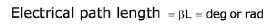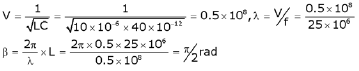Test: Electromagnetics - 2 - Question 13

A transmission line is lossless and is 25 m long. It is terminated with a load of zL =40+j30Ω at a frequency of 10 MHz. The inductance and capacitance of the line are L - 300nH/m, C = 40pF/m.

Q.

Find β

Detailed Solution for Test: Electromagnetics - 2 - Question 13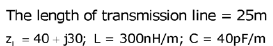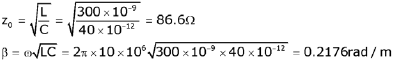Test: Electromagnetics - 2 - Question 14

A transmission line is lossless and is 25 m long. It is terminated with a load of zL =40+j30Ω at a frequency of 10 MHz. The inductance and capacitance of the line are L - 300nH/m, C = 40pF/m.

Q.

Find the input impedance at the mid-point of the line.

Detailed Solution for Test: Electromagnetics - 2 - Question 14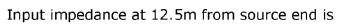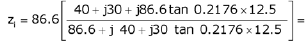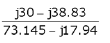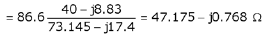Test: Electromagnetics - 2 - Question 15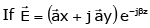then wave is said to be which one of the following,

Test: Electromagnetics - 2 - Question 16

An air filled rectangular wave guide has dimensions a = 2, b = 1 cm, the range of frequencies over which guide will operate in single mode is given by:

Detailed Solution for Test: Electromagnetics - 2 - Question 16

single mode propagation means only TE|0 mode will propagate.

Test: Electromagnetics - 2 - Question 17

For a rectangular waveguide of dimensions: a √3 × a (in cm) , if cut-off frequency of TE10 mode is 2GHz. What is the cut-off frequency for TM11 in waveguide?

Test: Electromagnetics - 2 - Question 18

If skin depth at 10 MHz for a conductor is 1/2 π meter then what is the phase velocity for this EM wave?

Detailed Solution for Test: Electromagnetics - 2 - Question 18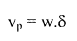Test: Electromagnetics - 2 - Question 19

The potential distribution is given by V = 10y4 + 2x3. If ε0 is permittivity of free space what is charge density (ρ) at (2, 0)

Test: Electromagnetics - 2 - Question 20

A short vertical grounded antenna is required to radiate at 1 MHz. The effective height of antenna is 302 meter then value of radiation resistance (approximately) is

Detailed Solution for Test: Electromagnetics - 2 - Question 20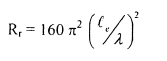## GATE ECE (Electronics) 2023 Mock Test Series

21 docs|263 tests
 Use Code STAYHOME200 and get INR 200 additional OFF Use Coupon Code
Information about Test: Electromagnetics - 2 Page
In this test you can find the Exam questions for Test: Electromagnetics - 2 solved & explained in the simplest way possible. Besides giving Questions and answers for Test: Electromagnetics - 2, EduRev gives you an ample number of Online tests for practice

## GATE ECE (Electronics) 2023 Mock Test Series

21 docs|263 tests